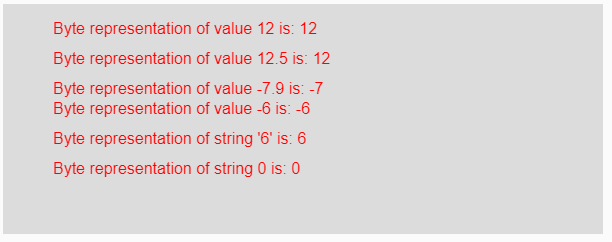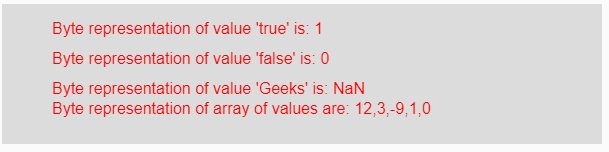# p5.js | byte() function

The byte() function in p5.js is used to convert the given string of number, number value or boolean into its byte representation. This byte number can only be whole number in between -128 to 127. The value outside of the range is converted into its corresponding byte representation.

Syntax:

`byte(Value)`

Parameters: This function accepts single parameter Value which is to be converted into its byte representation. This value might be integer, float, string, boolean, negative or positive value and array of values.

Return Value: It returns the converted byte representation.

Below program illustrates the byte() function in p5.js:

Example 1: This example uses byte() function to convert input elements into its byte representation.

 `function` `setup() {  ` `  `  `    ``// Creating Canvas size ` `    ``createCanvas(600, 230);  ` `}  ` `  `  `function` `draw() {  ` `      `  `    ``// Set the background color  ` `    ``background(220);  ` `    `  `    ``// Initializing some values ` `    ``let Value1 = 12; ` `    ``let Value2 = 12.5; ` `    ``let Value3 = -7.9; ` `    ``let Value4 = -6;  ` `    ``let Value5 = ``"6"``; ` `    ``let Value6 = 0; ` `    `  `    ``// Calling to boolean() function. ` `    ``let A = byte(Value1); ` `    ``let B = byte(Value2); ` `    ``let C = byte(Value3); ` `    ``let D = byte(Value4); ` `    ``let E = byte(Value5); ` `    ``let F = byte(Value6); ` `      `  `    ``// Set the size of text  ` `    ``textSize(16);  ` `      `  `    ``// Set the text color  ` `    ``fill(color(``'red'``));  ` `    `  `    ``// Getting byte representation ` `    ``text(``"Byte representation of value 12 is: "` `+ A, 50, 30); ` `    ``text(``"Byte representation of value 12.5 is: "` `+ B, 50, 60); ` `    ``text(``"Byte representation of value -7.9 is: "` `+ C, 50, 90); ` `    ``text(``"Byte representation of value -6 is: "` `+ D, 50, 110); ` `    ``text(``"Byte representation of string '6' is: "` `+ E, 50, 140); ` `    ``text(``"Byte representation of string 0 is: "` `+ F, 50, 170); ` `}  `

Output:Example 2: This example uses byte() function to convert input elements into its byte representation.

 `function` `setup() {  ` `  `  `    ``// Creating Canvas size ` `    ``createCanvas(600, 140);  ` `}  ` `  `  `function` `draw() {  ` `      `  `    ``// Set the background color  ` `    ``background(220);  ` `    `  `    ``// Initializing some values ` `    ``let Value1 = ``true``; ` `    ``let Value2 = ``false``; ` `    ``let Value3 = ``"Geeks"``; ` `    ``let Value4 = [12, 3.6, -9.8, ``true``, ``false``]; ` `    `  `    ``// Calling to byte() function. ` `    ``let A = byte(Value1); ` `    ``let B = byte(Value2); ` `    ``let C = byte(Value3); ` `    ``let D = byte(Value4); ` `      `  `    ``// Set the size of text  ` `    ``textSize(16);  ` `      `  `    ``// Set the text color  ` `    ``fill(color(``'red'``));  ` `    `  `    ``// Getting byte representation ` `    ``text(``"Byte representation of value 'true' is: "` `+ A, 50, 30); ` `    ``text(``"Byte representation of value 'false' is: "` `+ B, 50, 60); ` `    ``text(``"Byte representation of value 'Geeks' is: "` `+ C, 50, 90); ` `    ``text(``"Byte representation of array of values are: "` `+ D, 50, 110); ` `}     `

Output:Note: From the above examples, if the parameter is any fractional value then its output will be its integer equivalent i.e, a whole number between its range and string should be of number otherwise it gives output NaN i.e, not a number.

Reference: https://p5js.org/reference/#/p5/byteMy Personal Notes arrow_drop_upCheck out this Author's contributed articles.

If you like GeeksforGeeks and would like to contribute, you can also write an article using contribute.geeksforgeeks.org or mail your article to contribute@geeksforgeeks.org. See your article appearing on the GeeksforGeeks main page and help other Geeks.

Please Improve this article if you find anything incorrect by clicking on the "Improve Article" button below.

Article Tags :

Be the First to upvote.

Please write to us at contribute@geeksforgeeks.org to report any issue with the above content.